# Compact Wilkinsons

Click here to go to our general discussion of Wilkinson power splitters

On this page we describe three different techniques for shrinking the physical layout of a Wilkinson power splitter, but there are probably others we haven't considered so drop us a line if you run across one! We've also plotted the frequency response for each splitter, given a 50% shrink from the classic Wilkinson.

Compact Wilkinsons make up another class of Wilkinson power splitters. When would you want to use a compact Wilkinson? Suppose you wanted to use a Wilkinson power splitter at 1 GHz. The quarter-wave sections, laid out in microstrip on a soft substrate such as Duroid, would be a couple of inches long. You might not have room for them. Engineers have been trying different ways to shrink the Wilkinson since it was invented in 1960, but there are tradeoffs to each approach.

### Example 1: Webb splitter

Update December 2005: this Wilkinson variation was described in a 1981 issue of MicroWaves magazine,which later evolved into Microwaves and RF. The article was titled Power Divider/Combiners: Small Size, Big Specs, authored by Richard C. Webb. Don't bother searching on the MW&RF web site, they haven't made great stuff like this accessible to the teeming masses like we have. It took us some time to find a copy of the original article, but we have it now!

Webb's technique involves splitting each quarterwave arm into two smaller transmission lines, of unequal impedance. In the circuit shown below, the Wilkinson's quarter-wave sections of impedance 1.414xZ0 are replaced with two transmission lines in series, each of length "L1", measured in electrical degrees (90 degrees is one quarter wavelength). When L1 is 45 degrees, the Webb splitter is identical to the "classic" Wilkinson splitter (all impedances are 1.414x Z0). When L1 is shrunk to less than 45 degrees, the impedances of the lines are not equal, the impedance of the line closest to port 1 (Z0A) is lower than 1.414xZ0, while the other line impedance (Z0B) is higher than 1.414xZ0. A capacitor is used in the isolation network, this is the trick that makes it work.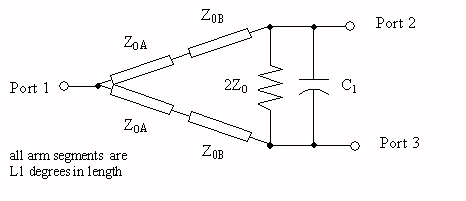Webb offered two variants of the splitter: one uses a parallel RC isolation network, and one uses a series RC network. Below are schematics we generated in Eagleware's Genesys, unfortunately we didn't use the "proper" nomenclature for the line impedances and lumped elements (maybe we'll fix this later).

Series isolation network (10 GHz capacitor value):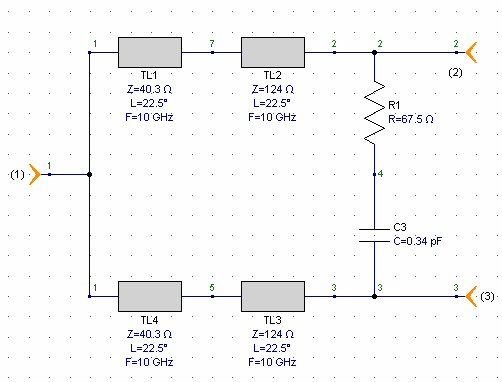Parallel isolation network (10 GHz capacitor value):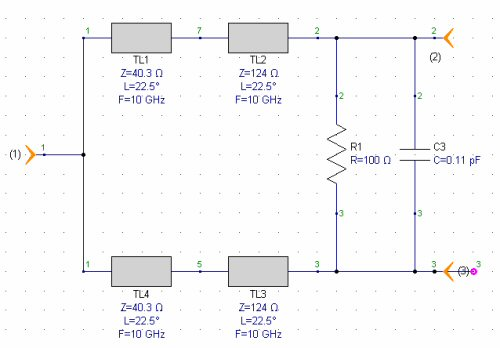Webb provided closed-form equations for line impedances and RC values for both variants, demonstrating math skills far beyond anything we possess! Below are the two solutions, we modified them so that you solve directly for capacitance in picofarads, instead of ohms of capacitive reactance. The angle "theta" is the electrical length in degrees (or radians if you use Excel). There was only one typo in the equations in the article, hopefully there are no typos here! (Oops, there was a typo, but it's fixed now thanks to Ramya).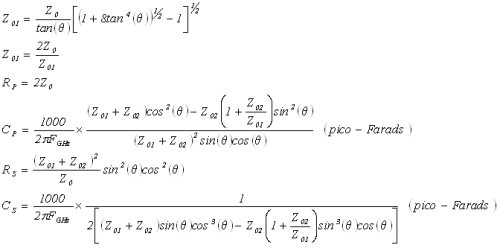Here are Webb's equations if you want to cut and paste them into Excel. Note that we replaced "theta" in the equations with "t" because we are too lazy to insert Greek characters in this case... Update December 18, 2006... Dave pointed out that the Excel equations for Cp and Cs were off by a factor of (-1). We think that they are all fixed now, but with that many parentheses it's still "buyer beware".

Z01=(Z0/tan(t))*((1+8*(tan(t)^4))^0.5-1)^0.5

Z02=(2*(Z0)^2/Z01)

Rp=2*Z0

Cp=((1000/(2*pi*f))*((Z01+Z02)*(cos(t)^2)-
Z02*(1+(Z02/Z01))*(sin(t))^2))/((Z01+Z02)^2*sin(t)*cos(t))

Rs=((Z01+Z02)^2/Z0)*((sin(t)^2)*(cos(t)^2))

Cs=(1000/(2*pi*f))*1/(2*(Z01+Z02)*(cos(t)^3)*sin(t)-
(Z02*(1+(Z02/Z01))*(sin(t)^3)*cos(t)))

Three things to note:

1. The line impedances Z01 and Z02 are the same for both series and parallel RC solutions.
2. Only the capacitor value is a function of design frequency.
3. In the parallel RC solution, the resistor value is unchanged from the classic Wilkinson at 2Z0.

Webb further offered a solution that would take into account the series inductance of the mounting pads of the lumped element RC network (we suggest that you model the isolation network an use an optimizer to finalize your design).

The two plots below shows the impedances of the lines, as well as the capacitor and resistor values (the first plot is for series RC, the second plot is for parallel RC). The compact Wilkinson has limitations: for L1=20 (length of arms compacted from 90 degrees to 40 total), the line impedance Z02 value of 140 ohms may be unattainably high. Note that when L1=45 degrees, the capacitor reaches zero value, and the line impedances are all 70.7 ohms, which is the solution for a regular fifty-ohm Wilkinson.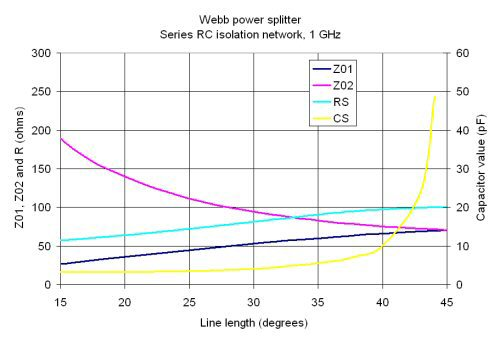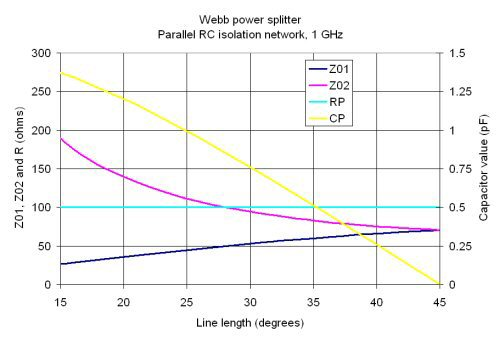Below we show frequency response plots for the series and parallel variations, given a 50% shrink (L1 is 22.5 degrees). They are quite similar, but the series RC version has better isolation bandwidth.

Webb splitter, L1=22.5 degrees, series isolation network, 10 GHz design: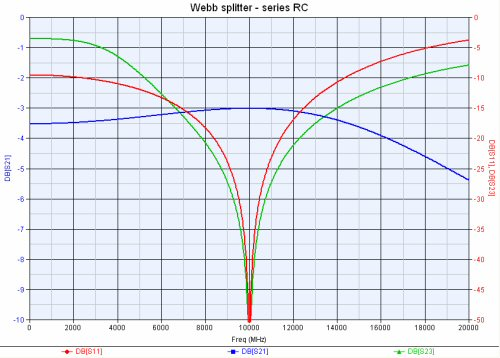Webb splitter, L1=22.5 degrees, parallel isolation network, 10 GHz design: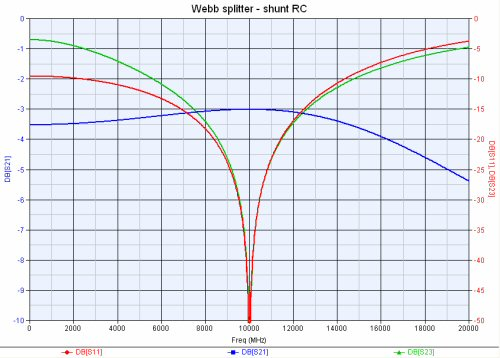The final thing to consider when using this compact Wilkinson is that your circuit will be at the mercy of the tolerance and temperature stability of capacitor C1. However, you could consider using open-circuit stubs in place of the capacitors for better uniformity.

### Example 2: Scardelletti splitter

This device was pointed out to us by Microwaves101 knifewinner Emily. It was described in an January 2002 IEEE article entitled Miniaturized Wilkinson Power Dividers using Capacitive Loading, by Scardelletti et al.

The technique used is to increase the line impedance of the arms, while loading the structure with capacitors C1 at the split ports and C2 at the common port (C2=2xC1). This is actually a pretty basic concept that we will eventually describe in our page on quarterwave tricks. Here' s a schematic we generated in Eagleware's Genesys (once again we ignored proper capacitor designators!)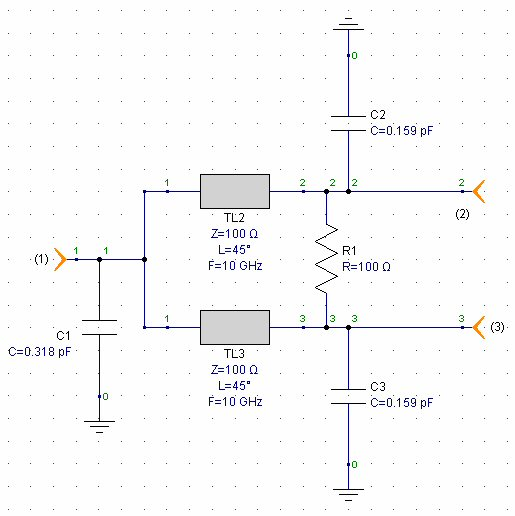In the referenced paper, equations are given for line impedance as a function of electrical length. Guess what? There's a mistake in the equation given for capacitance! We've fixed the mistake here: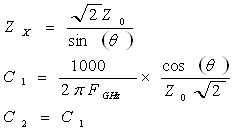The plot below shows the line impedances and shunt capacitor value as functions of line length in degrees, for this type of splitter in a fifty ohm system. When the line length is 90 degrees, the design reverts to a conventional Wilkinson coupler, with impedances 70.7 ohms and capacitors at zero value. The capacitor values given are for 1 GHz center frequency, if you want to scale them to other frequencies, merely divide them by the frequency you need (in GHz). Line impedances are NOT a function of frequency.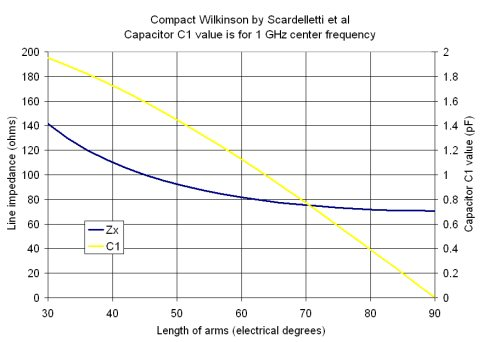Below is the frequency response of the Scardelletti splitter given a 50% shrink (arms are 45 degrees long). Looks like it doesn't achieve the same bandwidth as the Webb splitter, it rolls off quickly above the center frequency.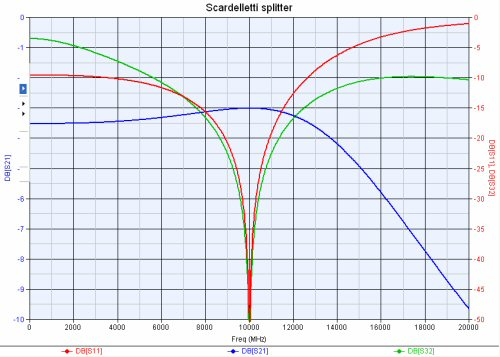### Example 3: Kang splitter

This technique was described by In-Ho Kang and Jin-San Park in their July 2003 Microwave Journal article entitled A Reduced-size Power Divider Using the Coupled Line Equivalent to a Lumped Inductor. It was pointed out to us on the Microwaves101 message board.

Update November 2006... we finally simulated the Kang splitter. Thanks to David from down under for sending us the article!

What Kang and Park figured out is that you can fake the quarterwave section using a coupled line that is shorted on two ports, with a pair of capacitors.

Before we describe Kang's splitter, let's look at a conventional Wilkinson realized using microstrip on 10 mil alumina. The next two images show the ADS schematic and its response: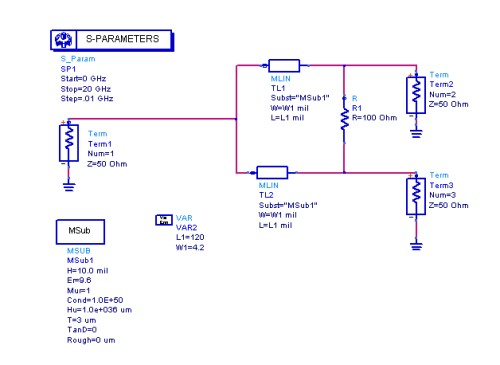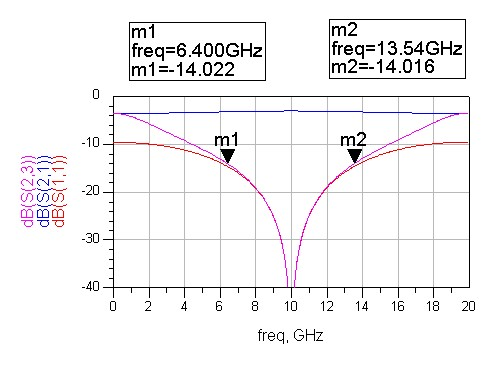Notice that a quarterwave section at 10 GHz is 120 mils.

The layout below represents about 10 minutes worth of work, trying to get the Kang splitter to behave at X-band, using 10 mil alumina. As you can see, the coupled line section is far less than a quarter wavelength, in this case it is closer to 1/16 wavelength. Tradeoffs that can be made include the coupling factor (spacing between the arms), length and impedance of the coupled section, and the capacitor values. Note that C1 and C2 could be combined into one capacitor of double the value, but we like the symmetry as it is shown.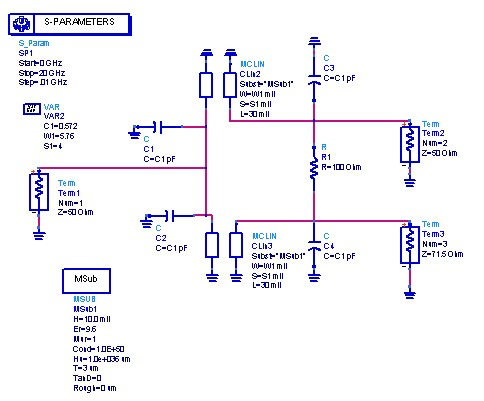Here's the response, it has NOT been fully optimized.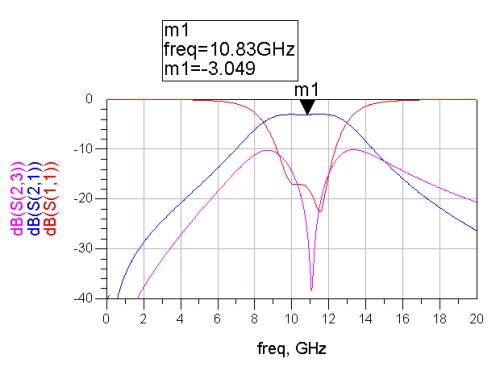More to come!

Author : Unknown Editor